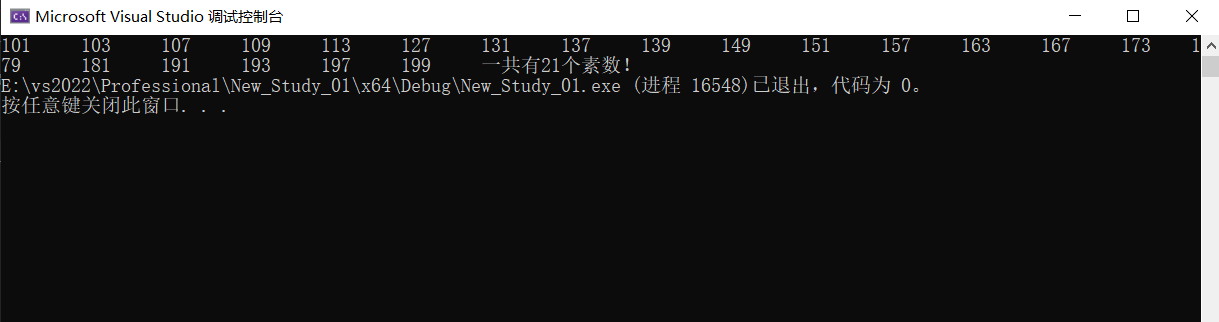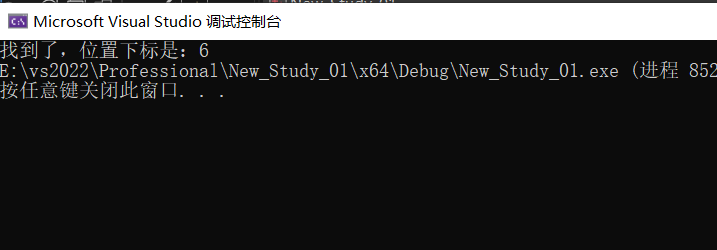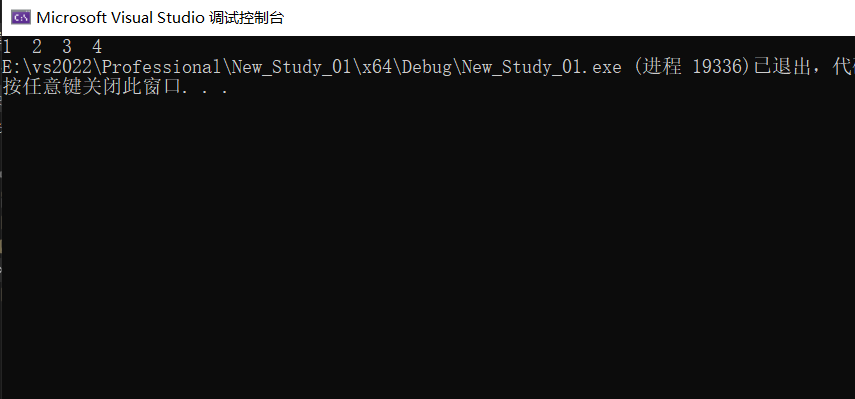﻿• 价格透明
• 信息保密
• 进度掌控
• 售后无忧# 手写C语言之函数操作-判断100-200内的素数-判断是否是闰年-二分查找函数实现-传址调用自增长函数实现（12）

### 目录

• 函数操作应用题
• 写一个函数可以判断一个数是不是素数。
• 写一个函数判断一年是不是闰年。
• 写一个函数，实现一个整形有序数组的二分查找。
• 写一个函数，每调用一次这个函数，就会将 num 的值增加1
• 每文一语### 函数操作应用题

1. 写一个函数可以判断一个数是不是素数。
2. 写一个函数判断一年是不是闰年。
3. 写一个函数，实现一个整形有序数组的二分查找。
4. 写一个函数，每调用一次这个函数，就会将 num 的值增加1。

### 写一个函数可以判断一个数是不是素数。

``````#include <stdio.h>
//定义函数名，注意因为有返回值，所以需要类型，其次传入一个整型变量
int is_prime(int x) {
int j = 0;
//素数的判断规则，从2到x-1进行尝试
for (j = 2; j < x; j++) {
if (x % j == 0) {
//取模操作符之后如果是0，就不是素数
return 0;
}
}
return 1;
}
int main() {
int i = 0;
int count = 0;
for (i = 100; i <= 200; i++) {
//调用判断函数
if (is_prime(i) == 1) {
printf("%d\t", i);
count++;
}

}
printf("一共有%d个素数！", count);
return 0;
}
``````注意，不建议将打印信息放入函数，因为函数是一个实现的功能，独立的，灵活性的！

### 写一个函数判断一年是不是闰年。

``````#include <stdio.h>

int is_leap_yar(int x) {
if (((x % 4 == 0) && (x % 100 != 0)) || (x % 400 == 0)) {
return 1;
}
else {
return 0;

}
}

int main() {

int y = 0;
for (y = 1000; y <= 2000; y++) {
//闰年函数的调用
if (is_leap_yar(y) == 1) {
printf("%d ", y);
}
}
return 0;
}

``````

### 写一个函数，实现一个整形有序数组的二分查找。

``````#include <stdio.h>
int serch(int a[], int k, int sz) {
int left = 0;
int right = sz - 1;
while (left <= right)
{
int mid = (left + right) / 2;
if (a[mid] > k) {
right = mid - 1;
}
else if (a[mid] < k) {
left = mid + 1;
}
else {
return mid;
}
}
return -1;
}
int main() {
int arr[] = { 1,2,3,4,5,6,7,8,9,10 };
int key = 7;
//找到就返回位置下标，找不到就返回-1
int sz = sizeof(arr) / sizeof(arr);

int ret = serch(arr, key, sz);
if (-1 == ret) {
printf("找不到\n");
}
else {
printf("找到了，位置下标是：%d", ret);
}
return 0;
}

``````### 写一个函数，每调用一次这个函数，就会将 num 的值增加1

``````#include <stdio.h>
(*p)++;//对其进行解引用，然后递增
}
int main() {
int num = 0;
printf("%d  ", num);
printf("%d  ", num);
printf("%d  ", num);
printf("%d  ", num);

return 0;
}
``````### 每文一语### 低价透明### 金牌服务### 信息保密